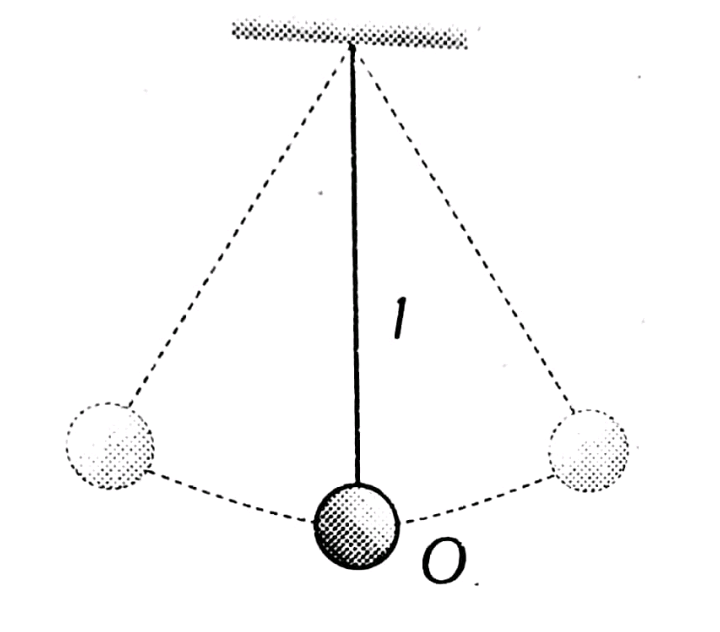Q

# I need help with The bob of a simple pendulum of mass m and total energy E will have maximum linear momentum equal to

The bob of a simple pendulum of mass m and total energy E will have maximum linear momentum equal to

• Option 1)

• Option 2)

• Option 3)

2mE

• Option 4)

mE2

92 Views

As we have learnt,

Simple Pendulum -

.

- whereinwhere E = Kinetic Energy, P = Momentum, m = Mass

So,

Option 1)

Option 2)

Option 3)

2mE

Option 4)

mE2

Exams
Articles
Questions